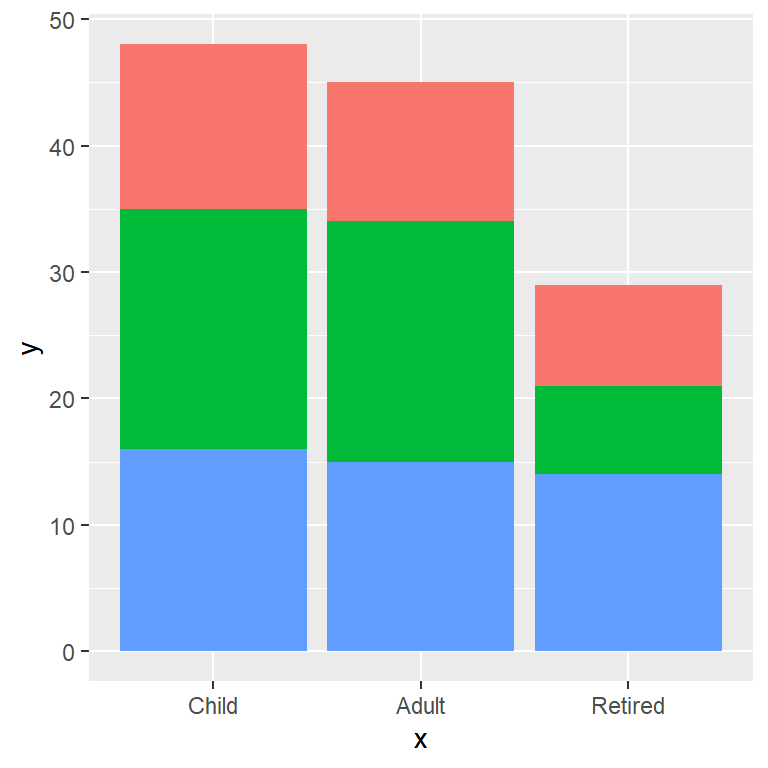# Stacked bar chart in ggplot2

## Sample data

The following data represents the answers to the question: “How many hours a day do you spend watching TV?”. The variable `x` represents the age of the person, `y` represents their answer and `group` represents their city. This toy data will be used in the examples below.

``````# Data
set.seed(1)

size = 50, replace = TRUE),
hours <- sample(1:4, size = 50, replace = TRUE)
city <- sample(c("A", "B", "C"),
size = 50, replace = TRUE)

df <- data.frame(x = age, y = hours, group = city)``````

## Basic stacked bar graph with `geom_bar`

stat = “count” (default)

If you use `geom_bar` with the default arguments you will need to pass only `x` or `y` to the `aes` in addition to the `fill`. The bar plot will display the stacked sum for each group of the variable.

``````# install.packages("ggplot2")
library(ggplot2)

ggplot(df, aes(x = x, fill = group)) +
geom_bar()``````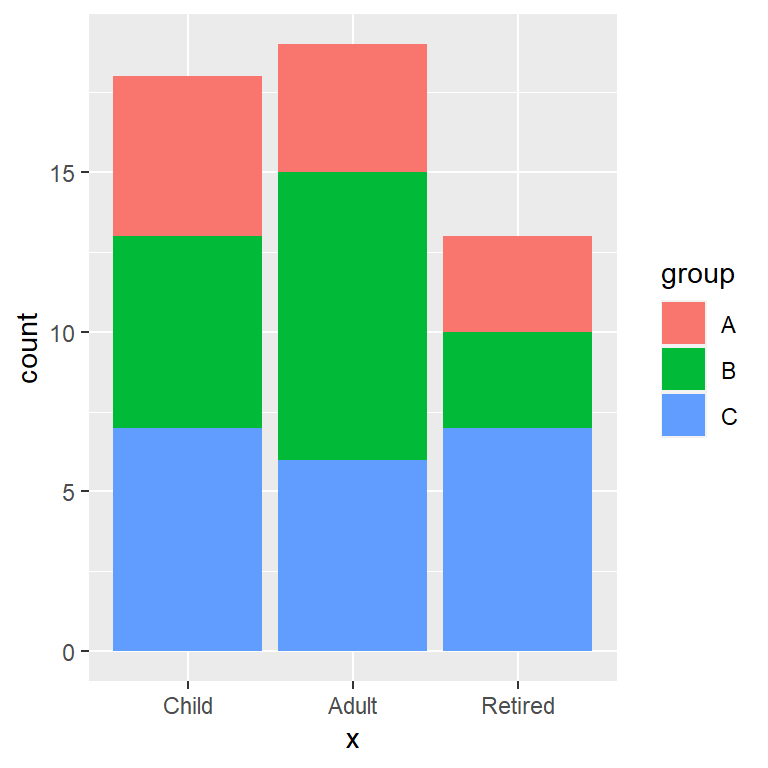stat = “identity”

Setting `stat = "identity"` you can create a stacked bar plot for multiple variables. In this scenario you can pass other variable to `aes`, representing the value or count of that variable.

``````# install.packages("ggplot2")
library(ggplot2)

ggplot(df, aes(x = x, y = y, fill = group)) +
geom_bar(stat = "identity")``````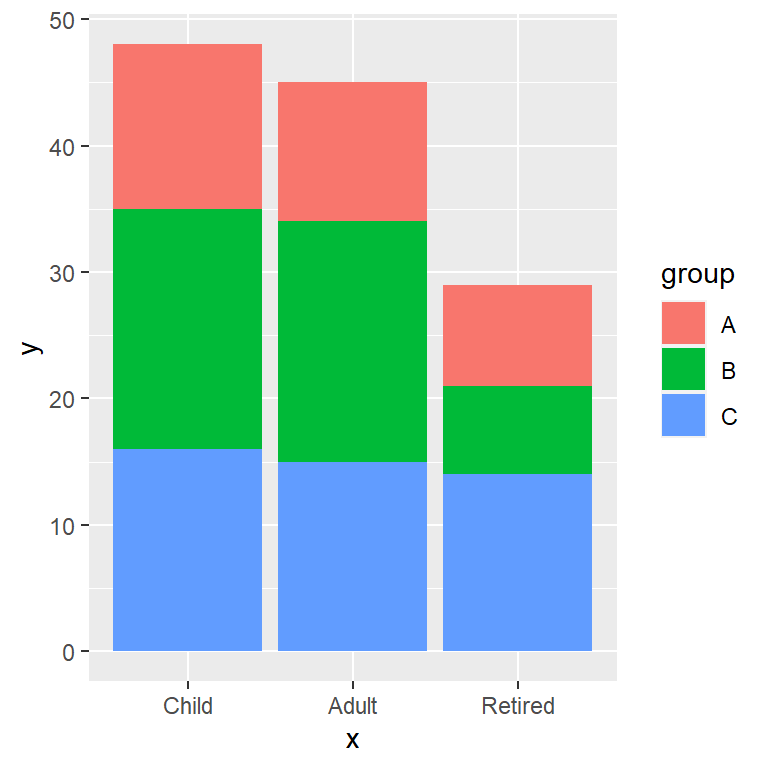## Bar fill and border colors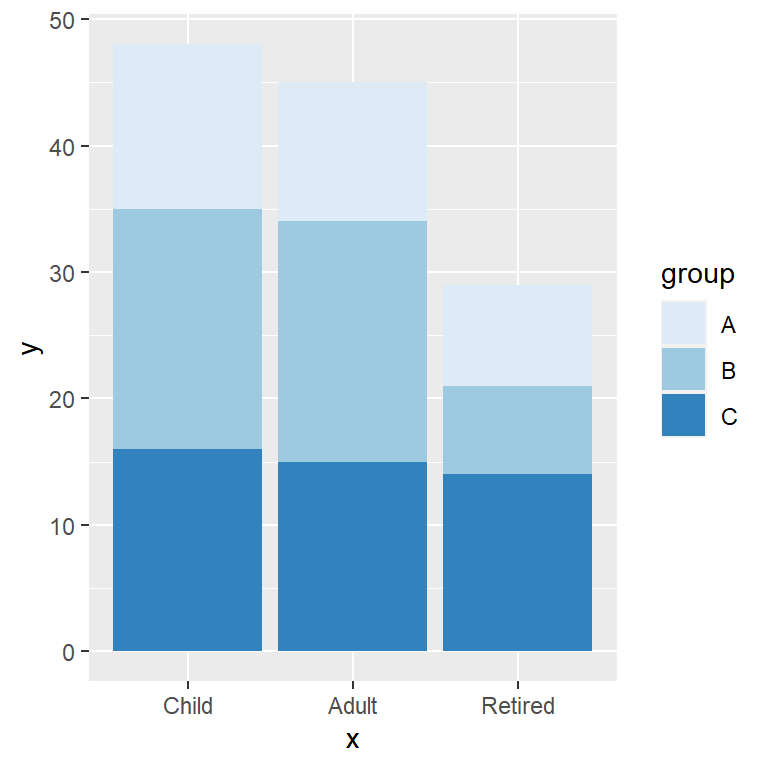Predefined palette

You can change the colors of the stacked bars with a predefined color palette, such as the ones provided by `scale_fill_brewer`.

``````# install.packages("ggplot2")
library(ggplot2)

ggplot(df, aes(x = x, y = y, fill = group)) +
geom_bar(stat = "identity") +
scale_fill_brewer()``````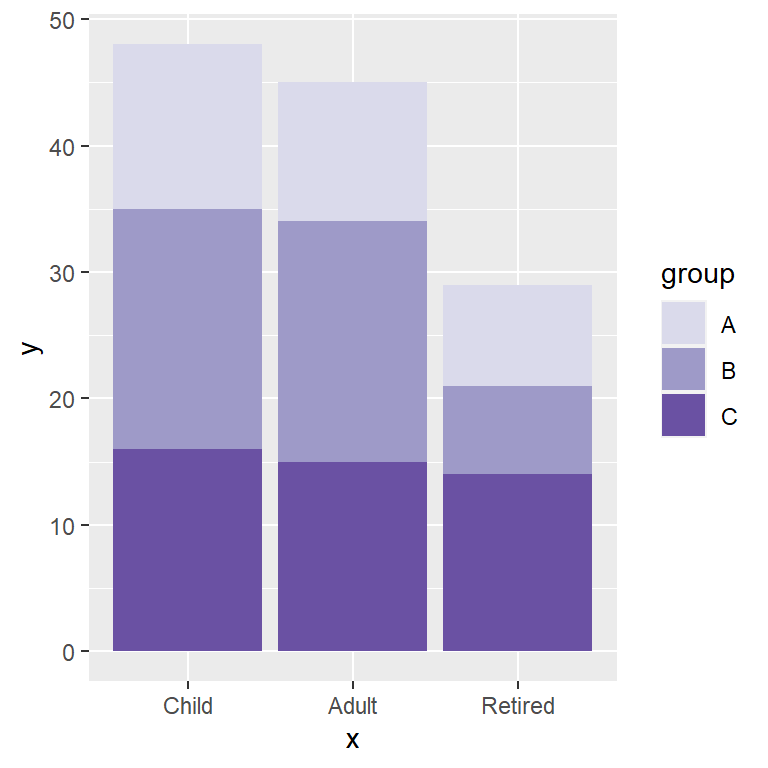Custom colors

If you prefer choossing each of the colors you can use `scale_fill_manual` and pass the vector of colors to the `values` argument.

``````# install.packages("ggplot2")
library(ggplot2)

ggplot(df, aes(x = x, y = y, fill = group)) +
geom_bar(stat = "identity") +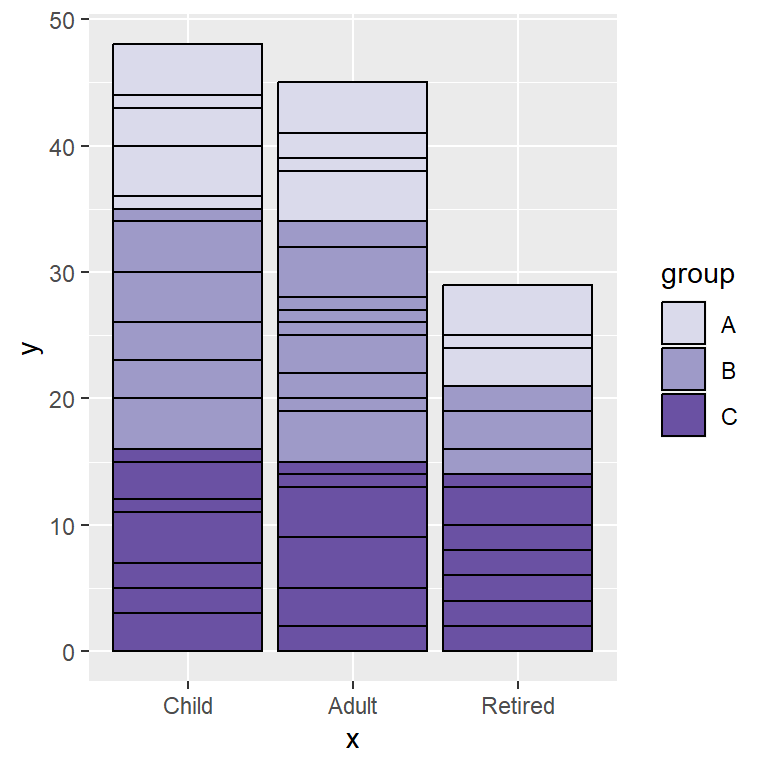Border color (stat = “identity”)

In case you are creating a bar graph with `stat = "identity"` you can add a border color passing a color to the `color` argument of `geom_bar`, but the border will outline all the bars representing the `y` variable.

``````# install.packages("ggplot2")
library(ggplot2)

ggplot(df, aes(x = x, y = y, fill = group)) +
geom_bar(stat = "identity", color = "black") +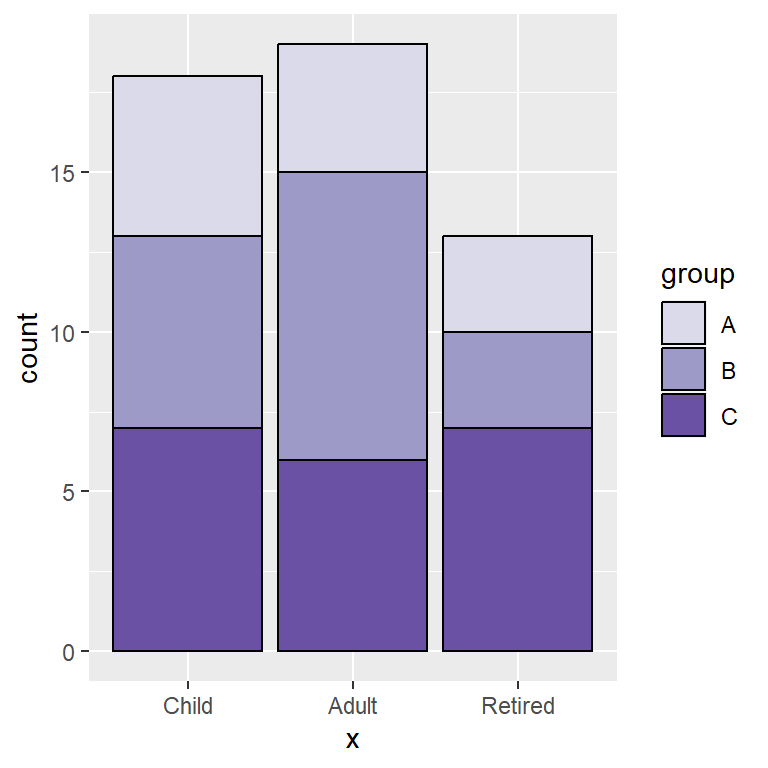Border color (stat = “count”)

If you create a stacked bar chart based on one variable the border will be placed around each bar, as there is no other variable involved.

``````# install.packages("ggplot2")
library(ggplot2)

ggplot(df, aes(x = x, fill = group)) +
geom_bar(color = "black") +

## Legend customization

Legend title

The default legend title of the bar chart corresponds to the name of the variable passed to `fill` and can be overridden with the code below.

``````# install.packages("ggplot2")
library(ggplot2)

ggplot(df, aes(x = x, y = y, fill = group)) +
geom_bar(stat = "identity") +
guides(fill = guide_legend(title = "Title"))``````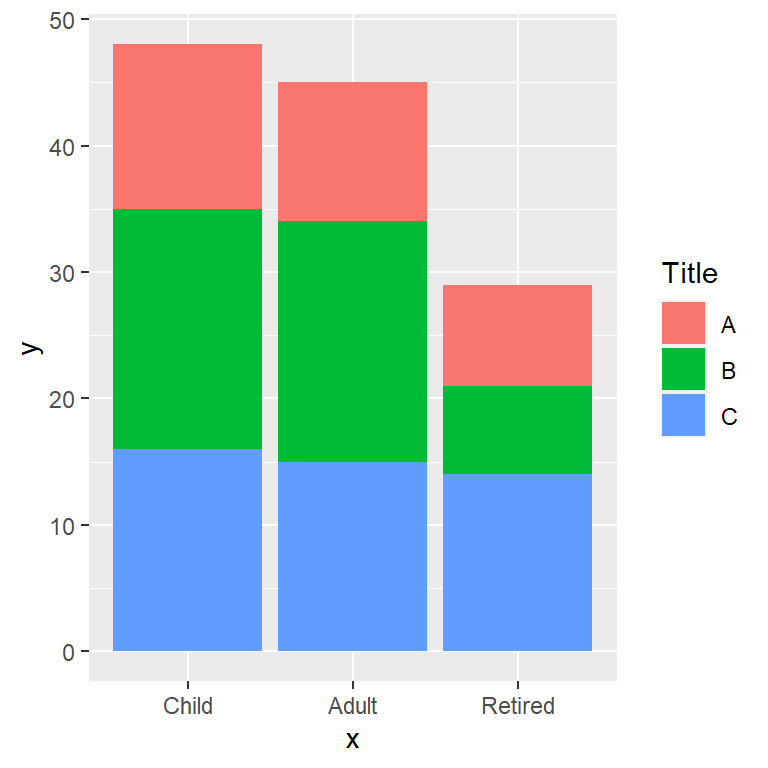Legend key labels

The key legend labels are the names of the categorical variable passed to `fill`. If you need to change these values you can use the `labels` argument of `sacale_fill_discrete` or `scale_fill_manual` if you are changing the fill colors.

``````# install.packages("ggplot2")
library(ggplot2)

ggplot(df, aes(x = x, y = y, fill = group)) +
geom_bar(stat = "identity") +
scale_fill_discrete(labels = c("G1", "G2", "G3"))``````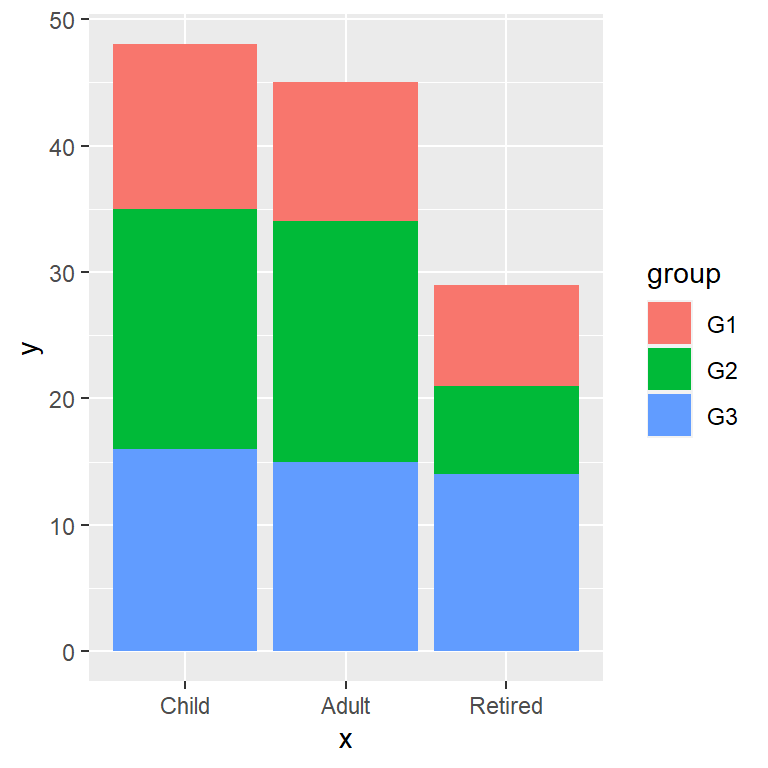Remove the legend

The legend can be removed setting the `legend.position` component of the `theme` function to `"none"`. Note that you can also change the position of the legend with this component.

``````# install.packages("ggplot2")
library(ggplot2)

ggplot(df, aes(x = x, y = y, fill = group)) +
geom_bar(stat = "identity") +
theme(legend.position = "none")``````